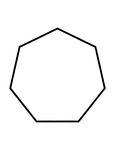### Flashcard of a polygon with seven equal sides

A flashcard featuring an illustration of a polygon with seven equal sides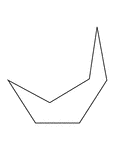### Irregular Concave Heptagon

Illustration of an irregular concave heptagon.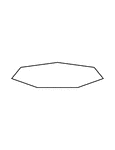### Irregular Convex Heptagon

Illustration of an irregular convex heptagon. This polygon has some symmetry.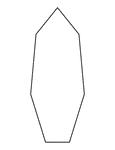### Irregular Convex Heptagon

Illustration of an irregular convex heptagon. This polygon has some symmetry.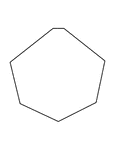### Irregular Convex Heptagon

Illustration of an irregular convex heptagon. This polygon has some symmetry.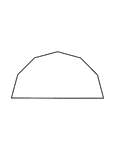### Irregular Convex Heptagon

Illustration of an irregular convex heptagon. This polygon has some symmetry.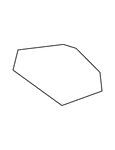### Irregular Convex Heptagon

Illustration of an irregular convex heptagon.### 7-sided Polygon

Polygon consisting of 7 sides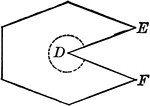### Concave Polygon

Illustration of a concave polygon. A concave polygon is a polygon of which tow or more sides, if produced,…### Fractions of 7-sided Polygon

1/7 of a 7 sided polygon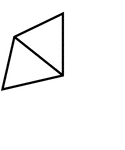### Fractions of 7-sided Polygon

2/7 of a 7 sided polygon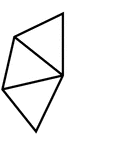### Fractions of 7-sided Polygon

3/7 of a 7 sided polygon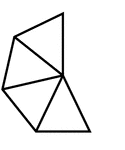### Fractions of 7-sided Polygon

4/7 of a 7 sided polygon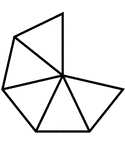### Fractions of 7-sided Polygon

5/7 of a 7 sided polygon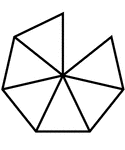### Fractions of 7-sided Polygon

6/7 of a 7 sided polygon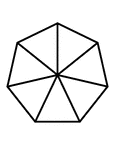### Fractions of 7-sided Polygon

7/7 of a 7 sided polygon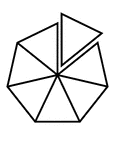### Fractions of 7-sided Polygon

7/7 of a 7 sided polygon with one piece detached